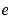MATLAB Function Referenceexpm

Matrix exponential

Syntax

• ```Y = expm(X)
```

Description

```Y = expm(X) ``` raises the constantto the matrix power `X`. The `expm` function produces complex results if `X` has nonpositive eigenvalues.

Use `exp` for the element-by-element exponential.

Algorithm

`expm` is a built-in function that uses the Padé approximation with scaling and squaring. You can see the coding of this algorithm in the `expm1` demo.

 Note    The `expmdemo1`, `expmdemo2`, and `expmdemo3` demos illustrate the use of Padé approximation, Taylor series approximation, and eigenvalues and eigenvectors, respectively, to compute the matrix exponential.References  and  describe and compare many algorithms for computing a matrix exponential. The built-in method, `expm`, is essentially method 3 of .

Examples

This example computes and compares the matrix exponential of `A` and the exponential of `A`.

• ```A = [1        1        0
0        0        2
0        0       -1 ];

```expm(A)
```ans =
2.7183   1.7183        1.0862
0        1.0000        1.2642
0             0        0.3679

```exp(A)
```ans =
2.7183        2.7183        1.0000
1.0000        1.0000        7.3891
1.0000        1.0000        0.3679
```

Notice that the diagonal elements of the two results are equal. This would be true for any triangular matrix. But the off-diagonal elements, including those below the diagonal, are different.

See Also

`exp`, `funm`, `logm`, `sqrtm`

References

  Golub, G. H. and C. F. Van Loan, Matrix Computation, p. 384, Johns Hopkins University Press, 1983.

  Moler, C. B. and C. F. Van Loan, "Nineteen Dubious Ways to Compute the Exponential of a Matrix," SIAM Review 20, 1979, pp. 801-836.

© 1994-2005 The MathWorks, Inc.